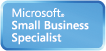Welcome Guest, you are in: Login

# MUST Creative Engineering Laboratory

### Technical Doc

Search the wiki
»

www.must.or.kr# Fourier transform

Modified on 2010/05/04 17:00 Categorized as Digital Image Processing
In mathematics, the Fourier transform (often abbreviated FT) is an operation that transforms one complex-valued function of a real variable into another. In such applications as signal processing, the domain of the original function is typically time and is accordingly called the time domain. That of the new function is frequency, and so the Fourier transform is often called the frequency domain representation of the original function. It describes which frequencies are present in the original function. This is in a similar spirit to the way that a chord of music can be described by notes that are being played. In effect, the Fourier transform decomposes a function into oscillatoryfunctions. The term Fourier transform refers both to the frequency domain representation of a function and to the process or formula that "transforms" one function into the other.

The Fourier transform and its generalizations are the subject of Fourier analysis. In this specific case, both the time and frequency domains are unbounded linear continua. It is possible to define the Fourier transform of a function of several variables, which is important for instance in the physical study of wave motion and optics. It is also possible to generalize the Fourier transform on discrete structures such as finite groups, efficient computation of which through a fast Fourier transform is essential for high-speed computing.